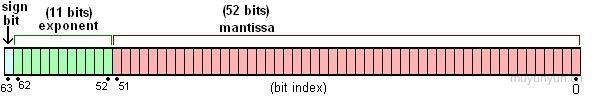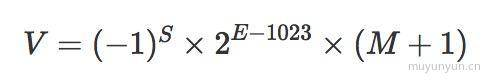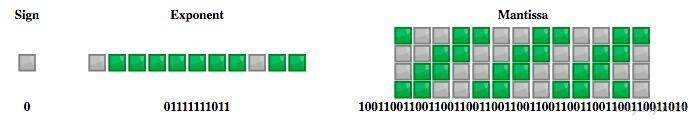Time Flying# 探寻 JavaScript 精度问题

### 十进制小数转为二进制小数方法

①. 针对整数部分 173, 采取`除 2 取余, 逆序排列`;

``````173 / 2 = 86 ... 1
86 / 2 = 43 ... 0
43 / 2 = 21 ... 1   ↑
21 / 2 = 10 ... 1   | 逆序排列
10 / 2 = 5 ... 0    |
5 / 2 = 2 ... 1     |
2 / 2 = 1 ... 0
1 / 2 = 0 ... 1``````

②. 针对小数部分 0.8125, 采用`乘 2 取整, 顺序排列`;

``````0.8125 * 2 = 1.625  |
0.625 * 2 = 1.25    | 顺序排列
0.25 * 2 = 0.5      |
0.5 * 2 = 1         ↓``````

③. 将前面两部的结果相加, 结果为 `10101101.1101`;

### 小心, 二进制小数丢失了精度！

``````0.1 * 2 = 0.2
0.2 * 2 = 0.4 // 注意这里
0.4 * 2 = 0.8
0.8 * 2 = 1.6
0.6 * 2 = 1.2
0.2 * 2 = 0.4 // 注意这里, 循环开始
0.4 * 2 = 0.8
0.8 * 2 = 1.6
0.6 * 2 = 1.2
...``````

### 推导 0.1 + 0.2 为何等于 0.30000000000000004• sign(符号): 占 1 bit, 0 表示正, 1 表示负;
• exponent(指数): 占 11 bit, 表示范围;
• mantissa(尾数): 占 52 bit, 表示精度, 多出的末尾如果是 1 需要进位;`0.1` 转化为二进制为 `0.0001100110011...`, 用科学计数法表示为 `1.100110011... x 2^(-4)`, 根据上述公式, `S``0`(1 bit), `E``-4 + 1023`, 对应的二进制为 `01111111011`(11 bit), `M``1001100110011001100110011001100110011001100110011010`(52 bit, 另外注意末尾的进位), `0.1` 的存储示意图如下:`0.1 + 0.2` 即 2^(-4) x 1.1001100110011001100110011001100110011001100110011010 与 2^(-3) x 1.1001100110011001100110011001100110011001100110011010 之和

``````// 计算过程
0.00011001100110011001100110011001100110011001100110011010
0.0011001100110011001100110011001100110011001100110011010

// 相加得
0.01001100110011001100110011001100110011001100110011001110``````

`0.01001100110011001100110011001100110011001100110011001110` 转化为十进制就是 `0.30000000000000004`。验证完成!

### JavaScript 的最大安全数是如何来的

``Math.pow(2, 53) === Math.pow(2, 53) + 1 // true``

`Math.pow(2, 53)` 竟然与 `Math.pow(2, 53) + 1` 相等！这是因为 Math.pow(2, 53) + 1 已经超过了尾数的精度限制(53 bit), 在这个例子中 `Math.pow(2, 53)``Math.pow(2, 53) + 1` 对应了同一个双精度浮点数。所以 `Math.pow(2, 53)` 就不是安全数了。

### 业务中碰到的精度问题以及解决方案

1.针对大数的整数可以考虑使用 BigInt 类型(目前在 stage 3 stage4 阶段)；

2.使用 bigNumber, 它的思想是转化成 string 进行处理, 这种方式对性能有一定影响;

3.可以考虑使用 long.js, 它的思想是将长度为 64 位的 long 类型值转化成两个精度为 32 位的双精度类型的值。

4.针对小数的话可以使用 number-precision, 该库将小数转为整数后再作处理;